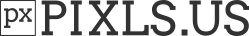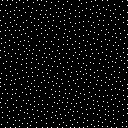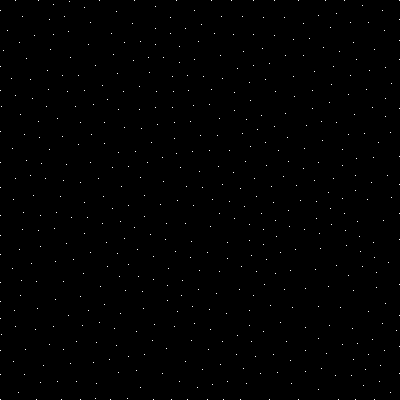# G'MIC exercises

Good example thanks, I’ve not yet used macros in the math parser! It’s obviously a commonly required algorithm type, therefore very useful to knowEdit: should’ve added, yes it’s random single elements being removed so this fits the case perfectly.

I’ll write one version that stores the data in an image stack instead, just to show it is as easy. As we can manage several images at the same time, this means having multiple dynamic lists is possible.

Here is a version with an image rather than a vector :

``````test :

i[list] 1,1,1,3,-1  # List of RGB colors

eval "

# Macro that inserts a new element 'elt' into the image #ind, at index 'pos' ('pos' must be in [0,siz_list]).
insert(ind,siz_list,elt,pos) = (
_pos = pos;
if (_pos<=siz_list,
siz_list>=w(#ind)?resize(#ind,2*w(#ind)+1,1,1,s#ind,0);
for (k = 0, k<s#ind, ++k, copy(i(#ind,_pos+1,0,0,k),i(#ind,_pos,0,0,k),siz_list - _pos));
I[#ind,_pos] = elt;
++#siz_list;
);
);

# Macro that removes an element from the image #ind, located at index 'pos' ('pos' must be in [0,siz_list]).
remove(ind,siz_list,pos) = (
_pos = pos;
if (_pos<=siz_list,
--#siz_list;
for (k = 0, k<s#ind, ++k, copy(I(#ind,_pos,0,0,k),I(#ind,_pos+1,0,0,k),siz_list - _pos));
I[#ind,siz_list] = -1;
)
);

siz_list = 0;

echo('---- Initial state ------------');
print(#"\$list"); print(siz_list); # Empty list.

insert(#"\$list",siz_list,[ 0,128,0 ],0);
insert(#"\$list",siz_list,[ 64,0,0 ],0);
insert(#"\$list",siz_list,[ 0,0,255 ],2);

echo('---- After three insertions ---');
print(#"\$list"); print(siz_list); # List with three elements.

remove(#"\$list",siz_list,0);

echo('--- After one removal ---------');
print(#"\$list"); print(siz_list); # List with two elements.
"
``````
1 Like

No problem. Actually list and element manipulation were the first things I tried, with limited success, when I encountered G’MIC scripting (which was not that long ago; am still a relatively new user). If I stare at your discussions long enough, I might learn something. Still struggling to follow the math and code but it is fun nevertheless.PS All of you can make it up by looking into An Error Reduction Technique in Richardson-Lucy Deconvolution Method.Actually I already had a look, not certain whether it applies generally because it appears directed towards a particular form of noise. I suspect some of the maths will be outside what I know just now anyway, so much to learn!

Some news about the management of dynamic arrays in the math parser.
I’ve added new functions in command `math_lib` to ease handling this type of structure.

Basically, you first define a 1-column image with the number of channels you want (filled with `0`). It will be used to represent your dynamic array. One example is worth a thousand words :

``````test :

1,1,1,3  # A dynamic array of RGB colors (initially empty).

v -
eval \${-math_lib}"

print(dar_size(#0)); # Print number of elements -> '0'

# 500 insertions at random positions.
for (k = 0, k<500, ++k,
dar_insert(#0,u([255,255,255]),round(u(dar_size(#0))));
ext('w 200,100%,0');  # Display array
);

print(dar_size(#0)); # Print number of elements -> '500'

# 200 removal at random positions.
for (k = 0, k<200, ++k,
dar_remove(#0,round(u(dar_size(#0)-1)));
ext('w 200,100%,0'); # Display array
);

print(dar_size(#0)); # Print number of elements -> '300'

dar_insert(#0,[1,2,3],125);
print(I[#0,125]);
"
v +
``````

Maybe @garagecoder will be able to use those functions ?EDIT: How this internally work ?
The trick here is to store the current number of element in the image, at position `[w*h*d-1]`, and to have a image height that is always greater or equal than `dar_size() + 1`. The function `dar_insert()` resizes the image when needed, and the function `dar_remove()` also resizes to save memory usage.
The height of an image is not equal to the number of elements in your dynamic arrays, that’s why there is a function `dar_size()` to get this information.

Nice! I’ll certainly start with that, thanks. I have time today to begin writing it and that means I don’t have to worry about handling that part1 Like

Might as well re-hijack the thread because I thought of an idea which is a compromise regarding what David and I were discussing earlier about linear versus nonlinear parameters attached to sliders: float and integer sliders which display and output linear values but move nonlinearly from left to right with specified modes and powers. It could have various modes such as n^x and x^n. Also, now that I think about it, step options for both integer and float sliders.

1 Like

Well this poisson disk noise turned out tougher than I expectedMainly because of dealing with 1D to 3D and not knowing enough about the math parser. Had to redesign the algorithm itself… this version is the first attempt so needs optimised and probably still has bugs (there’s even debug code still in). But it’s cool you can watch it drawing it point by point!

``````gcd_poisson_disk : skip \${1=2},\${2=30}
#  input image to draw samples on
dim={d>1?3:h>1?2:1} cw={0.999*\$1/sqrt(\$dim)}
({[w,h,d,1]}) y. c  #  image dimensions vector
{[ceil(I/\$cw),-1]}  #  "accelerator" grid/cells
r 1,1,1,\$dim,-1  # keep only used dimensions in 
1,1,1,\$dim 1,1,1,1  #  samples list,  active list
{vector\$dim(2*ceil(sqrt(\$dim))+1)}  #  cell proximity kernel
f. "dot([x,y,z]-int([w/2,h/2,d/2]),[1,w#2,w#2*h#2])" y. c
nm dims nm grid nm samples nm active nm prox
-v -
eval \${-math_lib}"
const N = "\$dim";
const grid_cw = "\$cw";
const max_sample_attempts = \$2;
prox = I#5;
mag2(vec) = (dot(vec,vec));

dar_insert(#3,I#1,0);               # dummy sample to simplify bounds checks
dar_insert(#3,u(I#1),1);            # add initial sample to list
dar_insert(#4,1,0);                 # add its index to active list
I(#2,int(I[#3,1]/grid_cw)) = 1;     # add its index to grid cell
tr=0;
whiledo (dar_size(#4)>0,
R = int(u(dar_size(#4)-1e-4));    # choose a random active list index

P = i[#4,R];                      # get the index of that sample
T = I[#3,P];                      # position vector of that sample

for (attempts=0, attempts < max_sample_attempts, ++attempts,

dowhile (S=4*(u(vectorN(1))-0.5); M=mag2(S), M <= 1 || M > 4);

X = T + radius * S;             # potential sample from annulus around T
if (min(X)<0 || min(I[#1,0]-X)<0, continue()); # check within bounds

# check proximity of surrounding points
G = int(X/grid_cw);             # grid cell position vector
GI = dot(G,[1,w#2,w#2*h#2]);    # grid cell direct buffer index

for (K=0;rejected=0, K<size(prox), ++K,
V = i[#2,GI+prox[K]];         # sample index from grid to check
);

if (!rejected,
Q = dar_size(#3);               # sample found, get new index
dar_insert(#3,X,Q);             # insert into samples list
dar_insert(#4,Q,dar_size(#4));  # insert its index into active
I(#2,G) = Q;                    # insert its index into grid
I(#0,X)=1;                      # draw the point
break();
);
);

if (attempts == max_sample_attempts, dar_remove(#4,P));
++tr; if(tr%2==0,ext('w 400,400,1'));
);
" -v +
``````

using:
`gmic 128,128,1,1 gcd_poisson_disk 4`1 Like

Decided to add other preview options. There’s a issue though, preview timeout.

``````#@gui _
#@gui <i>Reptorian</i>
#@gui Modulus operations : fx_modulus, fx_modulus_preview(0)
#@gui : note = note("This filters applies modulo operation after arithmetic operation. Note: Joan Rake's Sawtoothers are the expanded version of this filter.")
#@gui : sep = separator()
#@gui : Multiply = float(1,0,32)
#@gui : sep = separator(), Preview type = choice("Full","Forward horizontal","Forward vertical","Backward horizontal","Backward vertical","Duplicate top","Duplicate left","Duplicate bottom","Duplicate right","Duplicate horizontal","Duplicate vertical","Checkered","Checkered inverse")
#@gui : sep = separator(), note = note("<small>Author : <i>Reptorian</i>.      Latest update : <i>2018/08/18</i>.</small>")
fx_modulo:
repeat \$! l[\$>] split_opacity l
mul \$1 add \$2 mod 256
endl a c endl done
fx_modulus_preview :
-gui_split_preview "-fx_modulus \${1--2}", \$-1``````

That really reminds me the kind of things you get with the farthest point sampling method.
The example below implements it:

``````do_fps :
v -

# Initialisation
400,400 noise. 0.01,2 !=. 0

# Furthest point sampling.
repeat 500 +distance. 1 eval "i(#0,xM,yM) = 1" rm. done

v +
``````

which generates this image:It’s a bit long to compute since a full distance function is computed before inserting each new point.

Ah yes that makes sense, it’s the exact same thing (random points a certain minimum distance apart) but a slightly different algorithm. In theory, once written properly, the one I’m working on should be faster due to using a proximity map. That depends a lot on whether I do a good job thoughI have the idea to re-update the distance function also only where it is necessary. I will be interesting to compare the computation times of the two approaches.
Good luck !Hahah! If this is to become a competition it’s a very lopsided one in your favour. But we all win anyway because we hopefully get a fast poisson disk noise from itI’ve almost something readyAny suggestion for a command name ? `noise_farther` maybe ?

Hmm farther is perhaps a little vague (farther than what?)… some suggestions to give you more ideas (keeping the prefix):

noise_distance
noise_mindistance
noise_disk
noise_sample
noise_sampling
noise_sphere (if you’re dealing with the 3d usage)

I’ll add more if I think of them

I found a really dumb mistake by me (which explains why it was so slow), I was removing the wrong index from the active sample list! It should say R not P:

`if (attempts == max_sample_attempts, dar_remove(#4,R));`

Now it’s much faster!

Here is my attempt:

``````#@cli noise_maxdist : _nb_points[%]>=0
#@cli : Add random noise points to selected image such that added points are set with the maximal possible distance.
#@cli : \$\$ 300,300 noise_maxdist 1% dilate_circ 5
noise_maxdist : check "\$1>=0"
e[^-1] "Add noise points to image\$?, with maximal possible distance."
v - repeat \$! l[\$>]
N={round(\${"is_percent \$1"}?whd*\$1:\$1)}
if \$N
100%,100%,100% +f. inf
if {d==1} # 2d version
eval "
# Init with random point.
i(#1,round(u([0,0],[w,h]-1))) = 1;
x = w/2; y = h/2; r = max(w,h);
const N1 = "\$N"-1;
for (n = 0, n<N1, ++n,
x0 = max(0,x - r); y0 = max(0,y - r);
x1 = min(w - 1,x + r); y1 = min(h - 1,y + r);
ext('+z[^0] ',vtos([x0,y0,x1,y1]),' distance.. 1 min[-2,-1] j .,',vtos([x0,y0]),' rm.');

# Find local maximum of distance function (faster than doing it globally, and improve randomization).
xc = round(u(w-1)); yc = round(u(h-1)); r2 = r*2;
x0 = max(0,xc - r2); y0 = max(0,yc - r2);
x1 = min(w - 1,xc + r2); y1 = min(h - 1,yc + r2);
ext('+z ',vtos([x0,y0,x1,y1]));
s = stats(#-1); x = x0 + s; y = y0 + s;
r = i(#2,x,y); ext('rm.');

# Put new point on found location.
i(#1,x,y) = 1;
)"
else # 3d version
eval "
# Init with random point.
i(#1,round(u([0,0,0],[w,h,d]-1))) = 1;
x = w/2; y = h/2; z = d/2; r = max(w,h,d);
const N1 = "\$N"-1;
for (n = 0, n<N1, ++n,
x0 = max(0,x - r); y0 = max(0,y - r); z0 = max(0,z - r);
x1 = min(w - 1,x + r); y1 = min(h - 1,y + r); z1 = min(d - 1,z + r);
ext('+z[^0] ',vtos([x0,y0,z0,x1,y1,z1]),' distance.. 1 min[-2,-1] j .,',vtos([x0,y0,z0]),' rm.');

# Find local maximum of distance function (faster than doing it globally, and improve randomization).
xc = round(u(w-1)); yc = round(u(h-1)); zc = round(u(d-1)); r2 = 2*r;
x0 = max(0,xc - r2); y0 = max(0,yc - r2); z0 = max(0,zc - r2);
x1 = min(w - 1,xc + r2); y1 = min(h - 1,yc + r2); z1 = min(d - 1,zc + r2);
ext('+z ',vtos([x0,y0,z0,x1,y1,z1]));
s = stats(#-1); x = x0 + s; y = y0 + s; z = z0 + s;
r = i(#2,x,y,z); ext('rm.');

# Put new point on found location.
i(#1,x,y,z) = 1;
)"
fi
f "init(val = vectors(iM==im?1:iM)); i#1?val:I" k
fi
endl done v +
``````

works in 2D and 3D (but slow in 3D).

Example of use:

``````\$ gmic sp lena,128 noise_maxdist 3%
``````But I think this is still a bit slow (and I’ve no ideas to speed it up right now).

1 Like

Yes, just tested your code, @garagecoder, and it looks like you won the challengeHah good jobs it’s not my algorithmI have several ideas to speed it up as well, so it could be very fast eventually…